# Machine Learning Project: Image Classification

In this article, an image classification task will be discussed:

Through the project, my team and I applied 3 models seeking the highest result accuracy. The models used are:

K-means clustering, Support Vector Machine (SVC), and Convolutional Neural Network (CNN). My teammates and I will break down a model each — this article will tackle upon core logic and processes behind the SVC model.

But first, let's briefly go over the task itself:

# Task Summary

Our goal was to apply 3 different models over images from this dataset. The dataset contains about 32.000 pictures of various facial expressions sized in 48x48. The data is split into 2 sub-sets: 11% for testing purposes and another 89% representing the training set.

All pictures within both sub-sets are labeled with one of 7 possible emotions. They are, namely: Angry, fear, happy, neutral, disgust, sad, surprise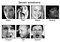Example of dataset images

The models would have to receive the training set as input in order to match as many images from the testing set with a corresponding correct label.

As the machine will have to choose either of 7 labels, the baseline (random-guess) accuracy is equal to 1/7 = 0.142857, or approximately 14.3%

Further on, this number will be used to assess the performance of each model

# K-means clustering

Performance: 27.9% accuracy… poor results, not far from random guessing.

This unsupervised clustering algorithm is the first one to discuss as it provides the poorest result out of all. The algorithm solves the task by allocating data points into clusters based on their similarities.

We were able to obtain the highest accuracy by setting the cluster amount to n=128. The graphs below represent the results achieved by this model. The left graph portrays the inertia of each cluster (i.e distance between data points within each cluster); the right graph visualizes the homogeneity and accuracy of each cluster.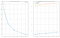K-means results

# SVC: Support Vector Machine

Performance: 36% accuracy… an improvement; x2.5 times better than random guessing.

The next model, SVC works by constructing a hyperplane in multidimensional space that allows it to separate different classes. The goal is to find the MMH (Maximum marginal hyperplane) that divides the dataset into classes the best way.

As our pictures are all sized 48x48 each total at 2304 dimensions what would make CSV code run an infinitely long time. To solve this issue, we applied PCA (Principal Component Analysis) to find how much an image can be shrunk to ease the work for the CVS.

Additionally, there is an important point for our dataset — our pictures are greyscaled. That means, that instead of 3 RGB values for each pixel we only have one: each is located within a range from 0 to 255 representing the pixel brightness.

The one last step before we can conduct the PCA is flattening. As implied by its name, this process will flatten the images converting each from a 48X48 matrix to one single vector with all pixel values one by one.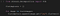Flattening the images

Finally, we can run the PCA and plot the results using the PCA module within the sklearn package: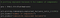Running the PCA & Plotting the results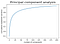PCA results

The result of the PCA, as showed above, allowed us to only around 100 components for each image, as it is enough to explain 90% of the variance between images. But before we continue, we determine the exact value of the amount of the component: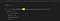Explaining 90% of the Variance

Indeed, 104 components are enough.

We decided to proceed with a component value of 150 to ensure higher accuracy. Now we can initiate the PCA with the determined value of components: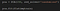Initializing the PCA

Following on, we approximate the images (i.e scale them down) to achieve 150 components per image.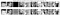Original images vs. 150-components images

Now, as we obtained the shrunk images we can finally run the CVS. After some struggles with input formatting (‘list’ object has no attribute ‘shape’ — that was a big headache), we run the model over the test images set!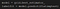Executing the model predictions

Here are the results: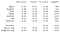CVS Results

As we obtained CVS chosen labels for the test dataset, we plot them to visualize the results. The red label stands for a wrong guess, black labels are correct predictions: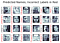CVS Results vizualized

# CNN: Convolutional Neural Network

Performance: 63% accuracy… Impressive improvement! An x4 times progress beyond the baseline.

The last, but clearly the best model we used was CNN, a neural network that uses an input layer, a number of hidden layers, and an output layer. It is commonly used for image classifications.

When we just started with CNN we experienced a lot of overfitting — our accuracy would continuously increase and achieve 99% within a couple of epochs, while validation accuracy (the measure testing against overfitting) would stay at a 20% level.

After a number of trial runs, we’ve been able to choose the layers resulting in the best performance. Here is the structure we used: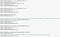CNN Model Layers

Further on, we run the model for 100 epochs and obtain great results: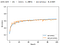CNN Model Results

As can be seen from the graph, the validation accuracy clearly follows the actual accuracy and both grow continuously. Hence, we solved the overfitting issue!

# Conclusion

Let's compare the performance of the 3 models used:

CNN — 63%

CVS — 36%

K-means — 27.9%

Clearly, CNN is a superior model for our specific task, as it is designed to classify images. The final result of 63% exceeds the baseline accuracy by more than 4 times, hence we can effectively claim our model performs well!

Thank you for the attention!

Erasmus University Student

## More from Matvei Fatin

Erasmus University Student